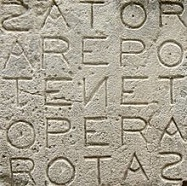# Palindromes in Matlab - working with indices

Palindromes are phrases, words, numbers or other sequences of characters that can be read the same way in either direction.

 In programming, they are used to learn to work with indices in a vector or an ascii string, so... there are many students with homeworks like this: How do I create this in Matlab? I need to create a program that reads 5 digit  integers and detects if it's a palindrome or not.

We can approach the problem in a number of ways, but let’s keep this solution simple. Since we are receiving the digits one-by-one, I propose to use a string input. Then, we utilize the buil-in function ‘num2str’ to transform the iteration number and include or concatenate that index in the string to be displayed to the user.To find an answer, I propose this naive code.

% I use these instructions to reset screen and memory
clear, clc

% We iterate five times
for i = 1 : 5

% Let's ask the user to enter digits one-by-one
str = ['Give me digit number ' num2str(i) ': '];
s(i) = input(str,
's');

% and we reverse the digits, starting by the end
p(6 - i) = s(i);
end

% Compare the entered string with the reversed one
if s == p

disp ('WOW! Those digits happen to be a palindrome!')
else
disp ('They are NOT a palindrome, sorry...')
end

On the other hand, there’s a function named ‘fliplr’ that exactly flips the array or matrix in left/right direction. We could have this slightly simplified code instead:

% We loop five times

for i = 1 : 5

% Let's input the digits
s(i) = input(['Give me digit number ' num2str(i) ': '],'s');
end

% Compare the entered string with the reversed one
if s == fliplr(s)

disp ('Way to go! Those digits are a palindrome!')
else
disp ('Nope, unfortunately they’re not, sorry...')
end

From 'Palindromes ' to home

From 'Palindromes ' to Matlab Examples

Credits:
M Disdero, CC BY-SA 3.0, via Wikimedia Commons